+关注继续查看

解剖SQLSERVER 第十五篇  SQLSERVER存储过程的源文本存放在哪里？（译）

http://improve.dk/where-does-sql-server-store-the-source-for-stored-procedures/

SET ANSI_NULLS ON
GO
SET QUOTED_IDENTIFIER ON
GO
-- =============================================
-- Author:
-- Create date:
-- Description:
-- =============================================
CREATE PROCEDURE XYZ
AS
BEGIN
-- SET NOCOUNT ON added to prevent extra result sets from
-- interfering with SELECT statements.
SET NOCOUNT ON;

-- Insert statements for procedure here
SELECT 'AABBCC' AS Output
END

select * from sys.procedures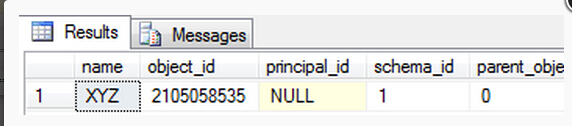select * from sys.sql_modules where object_id = 2105058535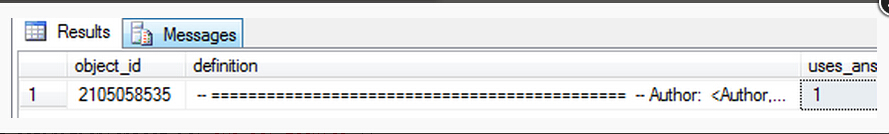sys.sql_modules ，sys.sql_modules 只是一个视图而不是系统表。我们看一下sys.sql_modules 视图是如何获取定义的：

select object_definition(object_id('sys.sql_modules'))
SELECT
object_id = o.id,
definition = Object_definition(o.id),
uses_ansi_nulls = Sysconv(bit, o.status & 0x40000), -- OBJMOD_ANSINULLS
uses_quoted_identifier = sysconv(bit, o.status & 0x80000),   -- OBJMOD_QUOTEDIDENT
is_schema_bound = sysconv(bit, o.status & 0x20000),    -- OBJMOD_SCHEMABOUND
uses_database_collation = sysconv(bit, o.status & 0x100000),  -- OBJMOD_USESDBCOLL
is_recompiled = sysconv(bit, o.status & 0x400000),     -- OBJMOD_NOCACHE
null_on_null_input = sysconv(bit, o.status & 0x200000),   -- OBJMOD_NULLONNULL
execute_as_principal_id = x.indepid
FROM
sys.sysschobjs o
LEFT JOIN
sys.syssingleobjrefs x ON x.depid = o.id AND x.class = 22 AND x.depsubid = 0 -- SRC_OBJEXECASOWNER
WHERE
o.pclass <> 100 AND
(
(o.type = 'TR' AND has_access('TR', o.id, o.pid, o.nsclass) = 1) OR
(type IN ('P','V','FN','IF','TF','RF','IS') AND has_access('CO', o.id) = 1) OR
(type IN ('R','D') AND o.pid = 0)
)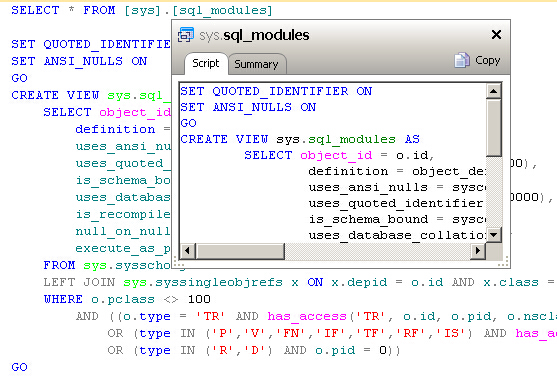select object_definition(object_id('object_definition'))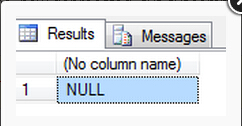SELECT
o.id AS id,
convert(smallint, case when o.type in ('P', 'RF') then 1 else 0 end) AS number,
s.colid,
s.status,
convert(varbinary(8000), s.text) AS ctext,
convert(smallint, 2 + 4 * (s.status & 1)) AS texttype,
convert(smallint, 0) AS language,
sysconv(bit, s.status & 1) AS encrypted,
sysconv(bit, 0) AS compressed,
s.text
FROM
sys.sysschobjs o
CROSS APPLY
OpenRowset(TABLE SQLSRC, o.id, 0) s
WHERE
o.nsclass = 0 AND
o.pclass = 1 AND
o.type IN ('C','D','P','R','V','X','FN','IF','TF','RF','IS','TR') AND
has_access('CO', o.id) = 1

UNION ALL

SELECT
c.object_id AS id,
convert(smallint, c.column_id) AS number,
s.colid,
s.status,
convert(varbinary(8000), s.text) AS ctext,
convert(smallint, 2 + 4 * (s.status & 1)) AS texttype,
convert(smallint, 0) AS language,
sysconv(bit, s.status & 1) AS encrypted,
sysconv(bit, 0) AS compressed,
s.text
FROM
sys.computed_columns c
CROSS APPLY
OpenRowset(TABLE SQLSRC, c.object_id, c.column_id) s

UNION ALL

SELECT
p.object_id AS id,
convert(smallint, p.procedure_number) AS number,
s.colid,
s.status,
convert(varbinary(8000), s.text) AS ctext,
convert(smallint, 2 + 4 * (s.status & 1)) AS texttype,
convert(smallint, 0) AS language,
sysconv(bit, s.status & 1) AS encrypted,
sysconv(bit, 0) AS compressed,
s.text
FROM
sys.numbered_procedures p
CROSS APPLY
OpenRowset(TABLE SQLSRC, p.object_id, p.procedure_number) s

UNION ALL

SELECT
o.id AS id,
convert(smallint, case when o.type in ('P', 'RF') then 1 else 0 end) AS number,
s.colid,
s.status,
convert(varbinary(8000), s.text) AS ctext,
convert(smallint, 2) AS texttype,
convert(smallint, 0) AS language,
sysconv(bit, 0) AS encrypted,
sysconv(bit, 0) AS compressed,
s.text
FROM
sys.sysobjrdb o
CROSS APPLY
OpenRowset(TABLE SQLSRC, o.id, 0) s
WHERE
db_id() = 1 AND
o.type IN ('P','V','X','FN','IF','TF')

HxD hex编辑器下载：

http://files.cnblogs.com/lyhabc/HxDhex%E7%BC%96%E8%BE%91%E5%99%A8.rar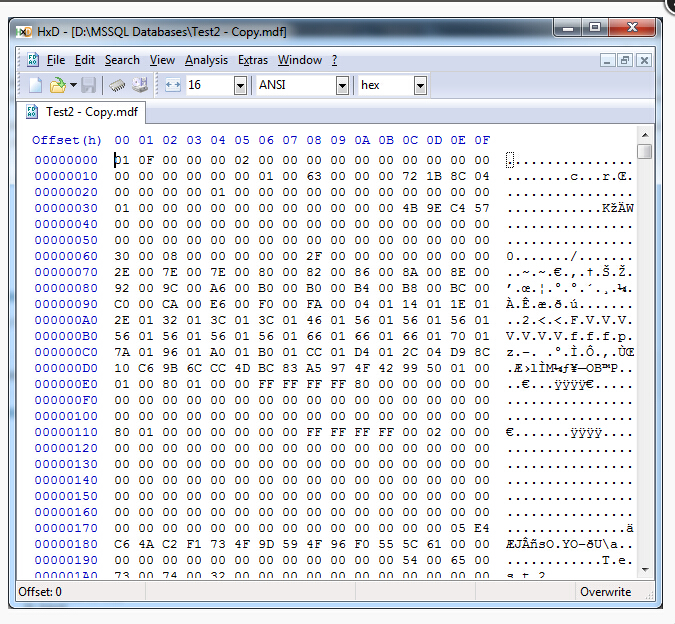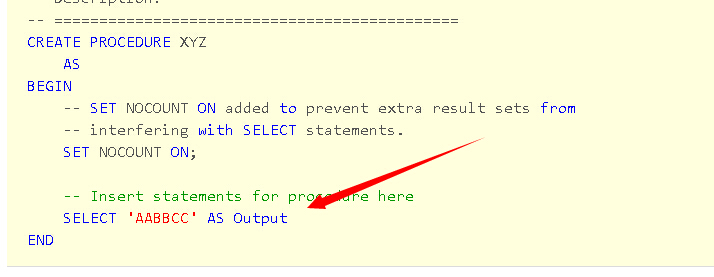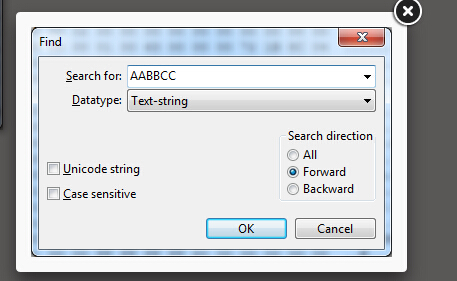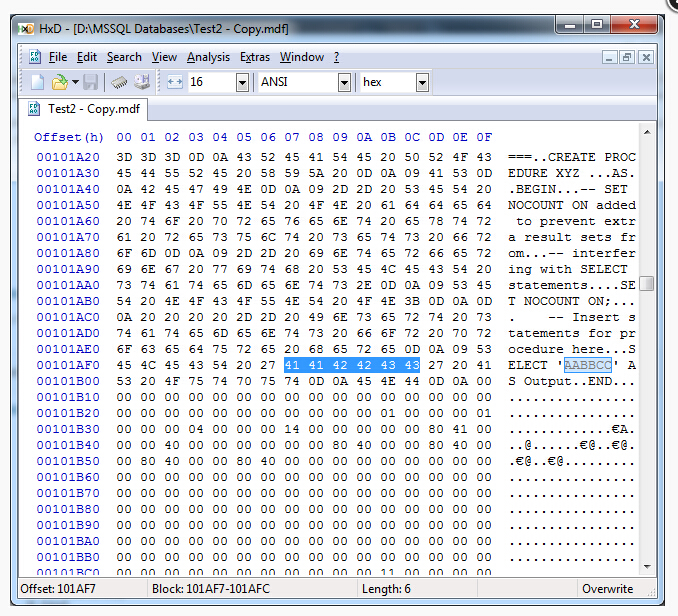01055472 / 8192 = 128

--只显示数据页面头
DBCC TRACEON (3604)
GO
DBCC PAGE(Test2, 1, 128, 0)
GO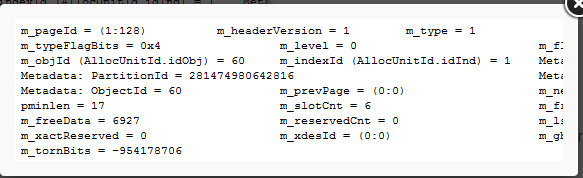select * from sys.sysobjects where id = 60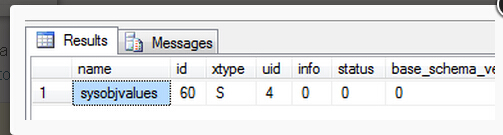select * from sys.sysobjvalues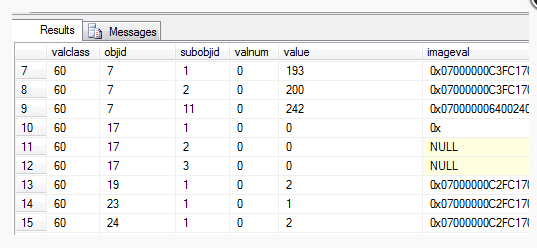select * from sys.sysobjvalues where objid = 2105058535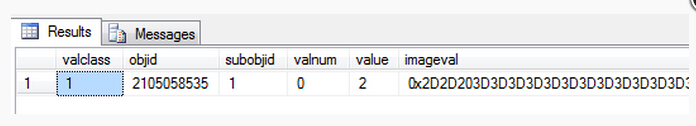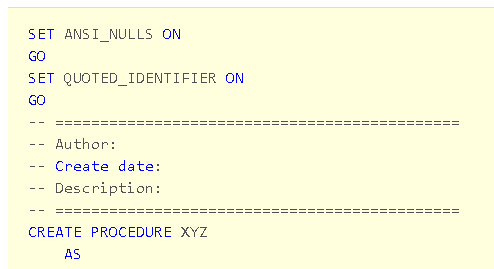select convert(varchar(max), imageval) from sys.sysobjvalues where objid = 2105058535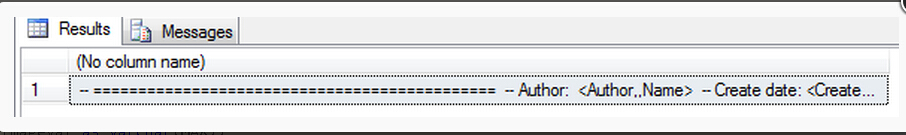select
p.name,
cast(v.imageval as varchar(MAX))
from
sys.procedures p
inner join
sys.sysobjvalues v on p.object_id = v.objid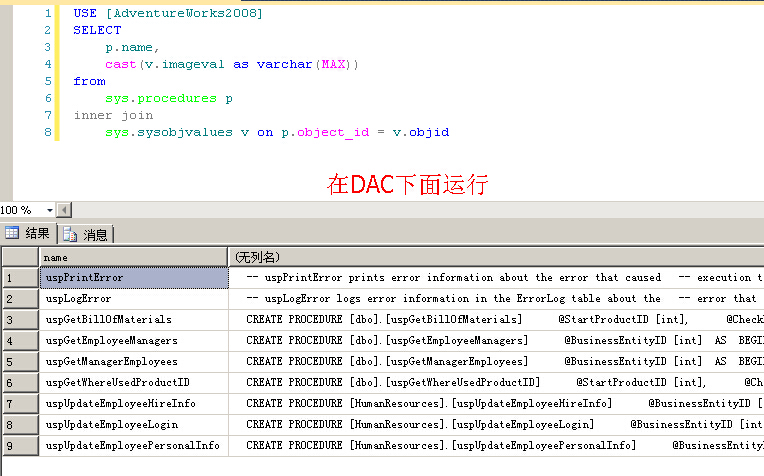10076 0sqlserver 通用分页存储过程

677 0806 0SQL SERVER 临时表导致存储过程重编译(recompile)的一些探讨

689 0【推荐】(SqlServer)不公开存储过程sp_Msforeachtable与sp_Msforeachdb详解

718 001.SQLServer性能优化之----强大的文件组----分盘存储

817 01030 013884 011888 06796 0

10427

2

《2021云上架构与运维峰会演讲合集》

《零基础CSS入门教程》

《零基础HTML入门教程》